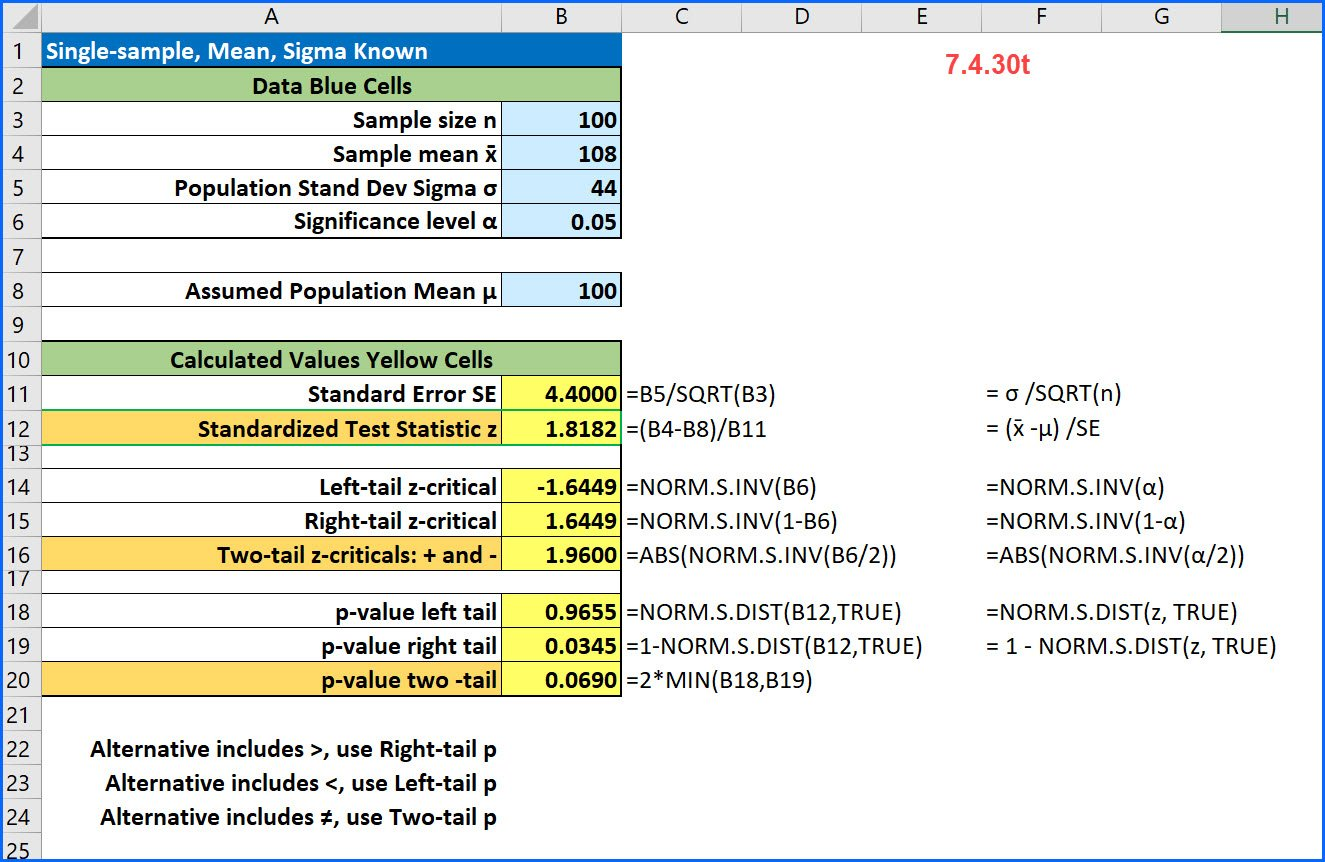Front### Tail of the Test: Interpreting Excel Data Analysis t-test output

Excel’s Data Analysis ToolPak has three tools for running tests of hypotheses using the t-distribution – t-tests. The output from the tools can be a bit confusing because, unlike other statistical software, these do not allow you to specify the “tail of the test” before you run the analysis. Here is how Microsoft explains how

This is the Excel solution using slightly different values:

### What is the Correlation Coefficient?

This is an excerpt from a longer, 12-minute video by Unbreaking America. I used it as an example of regression on a current event. I wonder how many Americans are data savvy enough to understand what Lawrence is showing them. I asked my students to identify the predictor variable and the response variable and toBUS 233### Single-sample z-test for the mean [7.4.30t]

Here is a common problem from intro stats: [7.4.30t] A random sample of 100 observations from a population with a standard deviation of 44 yielded a sample mean of 108. Test the null hypothesis that μ = 100 against the alternative that μ > 100 at an alpha of 0.05. Here because the alternative contains